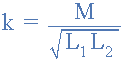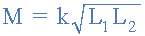# Mutual InductanceMutual Inductance is the interaction of one coils magnetic field on another coil as it induces a voltage in the adjacent coil.

In the previous tutorial we saw that an inductor generates an induced emf within itself as a result of the changing magnetic field around its own turns. When this emf is induced in the same circuit in which the current is changing this effect is called Self-induction, ( L ).

However, when the emf is induced into an adjacent coil situated within the same magnetic field, the emf is said to be induced magnetically, inductively or by Mutual induction, symbol ( M ). Then when two or more coils are magnetically linked together by a common magnetic flux they are said to have the property of Mutual Inductance.

Mutual Inductance is the basic operating principal of the transformer, motors, generators and any other electrical component that interacts with another magnetic field. Then we can define mutual induction as the current flowing in one coil that induces a voltage in an adjacent coil.

But mutual inductance can also be a bad thing as “stray” or “leakage” inductance from a coil can interfere with the operation of another adjacent component by means of electromagnetic induction, so some form of electrical screening to a ground potential may be required.

The amount of mutual inductance that links one coil to another depends very much on the relative positioning of the two coils. If one coil is positioned next to the other coil so that their physical distance apart is small, then nearly all of the magnetic flux generated by the first coil will interact with the coil turns of the second coil inducing a relatively large emf and therefore producing a large mutual inductance value.

Likewise, if the two coils are farther apart from each other or at different angles, the amount of induced magnetic flux from the first coil into the second will be weaker producing a much smaller induced emf and therefore a much smaller mutual inductance value. So the effect of mutual inductance is very much dependant upon the relative positions or spacing, ( S ) of the two coils and this is demonstrated below.

### Mutual Inductance between Coils

The mutual inductance that exists between the two coils can be greatly increased by positioning them on a common soft iron core or by increasing the number of turns of either coil as would be found in a transformer.

If the two coils are tightly wound one on top of the other over a common soft iron core unity coupling is said to exist between them as any losses due to the leakage of flux will be extremely small. Then assuming a perfect flux linkage between the two coils the mutual inductance that exists between them can be given as.

• Where:
•         µo is the permeability of free space (4.π.10-7)
•         µr is the relative permeability of the soft iron core
•         N is in the number of coil turns
•         A is in the cross-sectional area in m2
•         ℓ is the coils length in meters

## Mutual Induction

Here the current flowing in coil one, L1 sets up a magnetic field around itself with some of these magnetic field lines passing through coil two, L2 giving us mutual inductance. Coil one has a current of I1 and N1 turns while, coil two has N2 turns. Therefore, the mutual inductance, M12 of coil two that exists with respect to coil one depends on their position with respect to each other and is given as:

Likewise, the flux linking coil one, L1 when a current flows around coil two, L2 is exactly the same as the flux linking coil two when the same current flows around coil one above, then the mutual inductance of coil one with respect of coil two is defined as M21. This mutual inductance is true irrespective of the size, number of turns, relative position or orientation of the two coils. Because of this, we can write the mutual inductance between the two coils as: M12 = M21 = M.

Then we can see that self inductance characterises an inductor as a single circuit element, while mutual inductance signifies some form of magnetic coupling between two inductors or coils, depending on their distance and arrangement, an hopefully we remember from our tutorials on Electromagnets that the self inductance of each individual coil is given as:andBy cross-multiplying the two equations above, the mutual inductance, M that exists between the two coils can be expressed in terms of the self inductance of each coil.

giving us a final and more common expression for the mutual inductance between the two coils of:

### Mutual Inductance Between Coils

However, the above equation assumes zero flux leakage and 100% magnetic coupling between the two coils, L1 and L2. In reality there will always be some loss due to leakage and position, so the magnetic coupling between the two coils can never reach or exceed 100%, but can become very close to this value in some special inductive coils.

If some of the total magnetic flux links with the two coils, this amount of flux linkage can be defined as a fraction of the total possible flux linkage between the coils. This fractional value is called the coefficient of coupling and is given the letter k.

## Coupling Coefficient

Generally, the amount of inductive coupling that exists between the two coils is expressed as a fractional number between 0 and 1 instead of a percentage (%) value, where 0 indicates zero or no inductive coupling, and 1 indicating full or maximum inductive coupling.

In other words, if k = 1 the two coils are perfectly coupled, if k > 0.5 the two coils are said to be tightly coupled and if k < 0.5 the two coils are said to be loosely coupled. Then the equation above which assumes a perfect coupling can be modified to take into account this coefficient of coupling, k and is given as:

### Coupling Factor Between CoilsorWhen the coefficient of coupling, k is equal to 1, (unity) such that all the lines of flux of one coil cuts all of the turns of the second coil, that is the two coils are tightly coupled together, the resulting mutual inductance will be equal to the geometric mean of the two individual inductances of the coils.

Also when the inductances of the two coils are the same and equal, L1 is equal to L2, the mutual inductance that exists between the two coils will equal the value of one single coil as the square root of two equal values is the same as one single value as shown.

## Mutual Inductance Example No1

Two inductors whose self-inductances are given as 75mH and 55mH respectively, are positioned next to each other on a common magnetic core so that 75% of the lines of flux from the first coil are cutting the second coil. Calculate the total mutual inductance that exists between the two coils.

## Mutual Inductance Example No2

When two coils having inductances of 5H and 4H respectively were wound uniformly onto a non-magnetic core, it was found that their mutual inductance was 1.5H. Calculate the coupling coefficient that exists between.

In the next tutorial about Inductors, we look at connecting together Inductors in Series and the affect this combination has on the circuits mutual inductance, total inductance and their induced voltages.